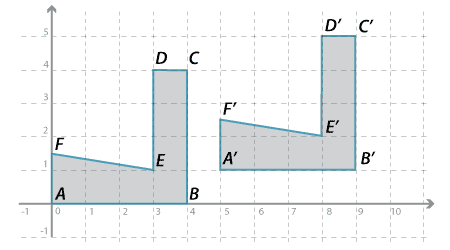### Congruence of plane figuresTwo objects like this are called congruent. The word 'congruent' comes from Latin and means 'in agreement' or 'in harmony'.

#### Definition

Two plane figures are called congruent if one figure can be moved so that it fits exactly on top of the other figure.

If two figures are congruent, such a movement can always be done by a sequence of translations, rotations and reflections. Reflect the first figure in any axis if it has the opposite parity to the second, then translate any point of the first figure to the matching point of the second figure, then rotate the first figure until it fits exactly on top of the second. Thus congruent figures can be defined in an alternative way that specifies the allowed transformations:Detailed description